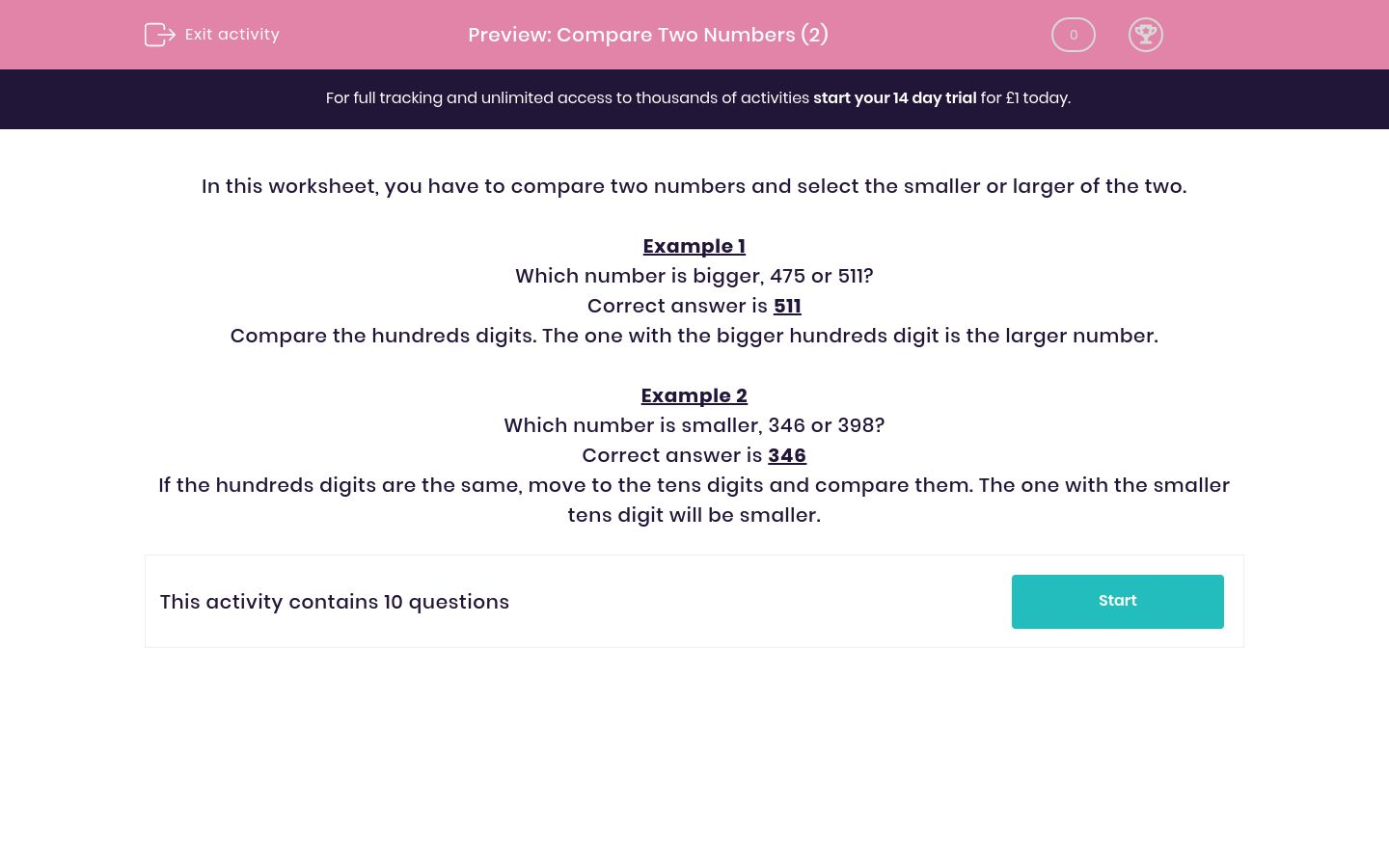# Compare Two Numbers (2)

In this worksheet, students compare two numbers and decide which is bigger or smaller.Key stage:  KS 2

Curriculum topic:   Maths and Numerical Reasoning

Curriculum subtopic:   Place Value

Difficulty level:### QUESTION 1 of 10

In this worksheet, you have to compare two numbers and select the smaller or larger of the two.

Example 1

Which number is bigger, 475 or 511?

Compare the hundreds digits. The one with the bigger hundreds digit is the larger number.

Example 2

Which number is smaller, 346 or 398?

If the hundreds digits are the same, move to the tens digits and compare them. The one with the smaller tens digit will be smaller.

Which number is less, 672 or 848?

672

848

Which number is more, 940 or 142?

940

142

Which number is more, 935 or 372?

935

372

Which number is less, 957 or 841?

957

841

Which number is smaller, 664 or 709?

664

709

Which number is smaller, 829 or 755?

829

755

Which number is smaller, 612 or 807?

612

807

Which number is smaller, 481 or 291?

481

291

Which number is more, 820 or 714?

820

714

Which number is bigger, 650 or 646?

650

646

• Question 1

Which number is less, 672 or 848?

672
• Question 2

Which number is more, 940 or 142?

940
• Question 3

Which number is more, 935 or 372?

935
• Question 4

Which number is less, 957 or 841?

841
• Question 5

Which number is smaller, 664 or 709?

664
• Question 6

Which number is smaller, 829 or 755?

755
• Question 7

Which number is smaller, 612 or 807?

612
• Question 8

Which number is smaller, 481 or 291?

291
• Question 9

Which number is more, 820 or 714?

820
• Question 10

Which number is bigger, 650 or 646?